# Sample method

(diff) ← Older revision | Latest revision (diff) | Newer revision → (diff)

A statistical method for the study of the general properties of a certain population of objects by studying the properties of only a sample (a part) of these objects. The mathematical theory of sample methods is based on two important sections of mathematical statistics — the theory of sampling from a finite population and the theory of sampling from an infinite population. The fundamental difference between the sampling theory for finite and infinite populations consists in the fact that in the former case the theory is usually applied to objects of a non-random, determined nature (for example, the number of defective articles in a given industrial batch of products is not a random variable: it is an unknown constant which must be estimated from the sampling data). In the latter case the theory is usually employed to study the properties of random objects (for example, to study the properties of continuously-distributed random experimental errors, each one of which may be interpreted, in principle, as the realization of one out of an infinite set of possible results).

Samples from finite populations and their theory form the base of methods in statistical quality control and are often employed in sociological studies. According to probability theory, the sample will correctly reproduce the properties of the population as a whole if the sampling is conducted at random, i.e. so that each one of the possible samples of a given sizeout of a population of size(the number of such samples is) has an equal chance of being selected in actual practice.

The method which is most often used in practice is sampling without replacement, the item already chosen not being returned to the population under study before taking the next items in the sample (for example, in drawing the winning lottery tickets, in statistical quality control and in long lasting demographic investigations). Sampling with replacement is usually employed in theoretical studies only (an example of this technique is the recording of the number of particles colliding with the container walls during a given period of time in the study of Brownian motion). If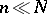, practically equivalent results are obtained from the two techniques.

The properties of the populations which are studied by the sample method may be qualitative and quantitative. In the former case the task of investigating the sample consists of finding the numberof items in the population having certain characteristics (e.g. during statistical control the parameter of interest is often the numberof defective items in a batch ofitems).is estimated by the ratio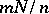, whereis the number of items displaying the characteristic under study in a sample of size. In the case of a quantitative characteristic the task consists of determining the mean value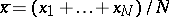of the population. The valueis estimated by means of the sample average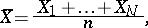where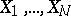are the numbers of quantities in the populations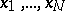under study which belong to the sample. From the mathematical point of view, the former situation is a special case of the latter, which occurs ifof the variables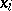are equal to one, while the remaining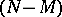are zero; in this situationand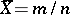.

In the mathematical theory of sample methods, estimating the mean value is the key operation, since this value forms the base of a quantitative description of the variability of the characteristic within the population; in fact, the variability of the characteristic is usually defined as the variance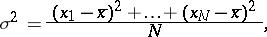which is the average of the squares of the deviations offrom the average value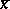. If a qualitative characteristic is studied, then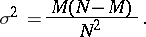The accuracies of the estimatesand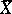are found from their varianceswhich are expressed, in terms of the variances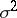of the finite population, as the ratios(in the case of sampling with replacement) and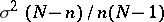(in the case of sampling without replacement). Since in many problems of practical interest the random variables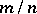androughly follow a normal distribution if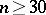, it follows that the deviations offromandfrom, with absolute values larger than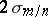and, respectively, may occur, on the average, in about one case in twenty.

More complete information about the distribution of a quantitative characteristic in a given population may be obtained from the empirical distribution of this characteristic in the sample.

## Contents

### Sampling from an infinite population.

It is usual in mathematical statistics to describe as a sample the results of given homogeneous observations (mostly independent ones) even through this differs from the concept of a sample from a finite population with or without replacement. Thus, the measurements of angles, which involve continuously-distributed random errors, would be denoted as a sample from an infinite population. It is assumed that it is possible, in principle, to carry out any desired number of such observations. The results obtained form a so-called sample from an infinite set of possible results, which is called the general aggregate. The concept of a general aggregate is neither logically unobjectionable nor indispensable. In solving practical problems, there is no need of the infinite general aggregate itself, but only of certain characteristics corresponding to it. From the point of view of probability theory, these characteristics are numerical or functional properties of a certain probability distribution, while the sample items are random variables subject to this distribution. Such an interpretation makes it possible to apply the general theory of statistical estimation to sample estimates. This is why, for example, in probability theory, when processing observations, the concept of an infinite general aggregate is replaced by the concept of a probability distribution involving unknown parameters. The results of the observations are treated as experimentally found values of the random variables subject to this distribution. The objective of the processing is to use the results of the observations to compute optimal (in some sense) statistical estimators for the unknown distribution parameters.

So far, the concern has been with sampling from one population of certain objects. In practice, however, sampling is often performed with several identical populations (e.g. in estimating the fraction of defective articles in several batches of finished industrial products). In such a situation the object of study is no longer a single number, but several unknown numbers. For instance, let each batch of the finished product containarticles, let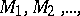be the numbers of defective articles in these batches, and let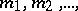be the corresponding numbers of defective articles found in samples of size. If the so-called principle of defect-free acceptance is accepted, the-th batch is delivered to the customer ifand is rejected otherwise. If it is assumed that the control of the articles involves their destruction, then the customer obtains a batch of size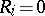(if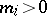) or a batch of size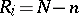containing(if) defective articles, the values of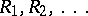(thus, their sum as well) being known, while the value of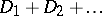is not known. The ratio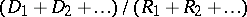is known as the fraction of passed defectives, and its mathematical expectationis known as the average fraction of passed defectives. The task of mathematical statistics is to estimatefrom the values ofwhich are determined using the sample method. If the valuesmay be treated as the realization of independent identically-distributed random variables with a known distribution law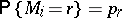, then, according to the Bayes formula, a statistical estimator of the average number of passed defective articles in the accepted batches can be expressed by the formulaand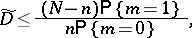where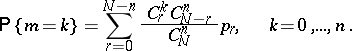For this reason the estimator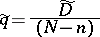of the average fraction of passed defectives in the accepted batches satisfies the inequality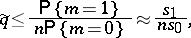where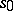is the number of accepted batches while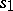is the number of defective batches the samples of which yielded exactly one defective article.

How to Cite This Entry:
Sample method. Encyclopedia of Mathematics. URL: http://encyclopediaofmath.org/index.php?title=Sample_method&oldid=16362
This article was adapted from an original article by L.N. Bol'shev (originator), which appeared in Encyclopedia of Mathematics - ISBN 1402006098. See original article